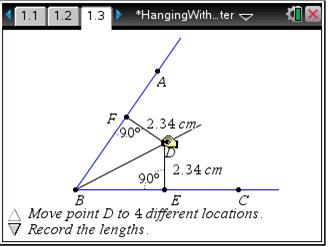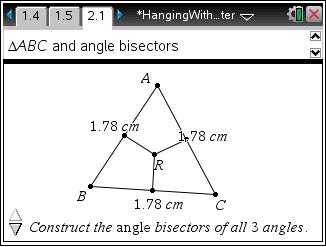# Activities

••• ##### Subject Area

• Math: Geometry: Analytic Geometry
• Math: Geometry: Triangles

• ##### Author9-12

15 Minutes

• ##### Device
•TI-Nspire™ CX/CX II
•TI-Nspire™ CX CAS/CX II CAS
• TI-Nspire™
• TI-Nspire™ CAS
• ##### Software

TI-Nspire™
TI-Nspire™ CAS

3.2

## Hanging with the Incenter

#### Activity Overview

In this activity, students will explore the angle bisector of the angles of a triangle. Students will discover that the angle bisectors are concurrent. The point of concurrency is the incenter. Students should discover the relationship between the type of triangle and the location of the point of concurrency.

#### Key Steps

•In this activity, students will explore the angle bisector. Students will discover that a point on the angle bisector is equidistant from the sides of the angle.

•Students will also explore the angle bisector of the angles of a triangle. Students will discover that the angle bisectors are concurrent. The point of concurrency is the incenter. Students should discover the relationship between the type of triangle and the location of the point of concurrency.